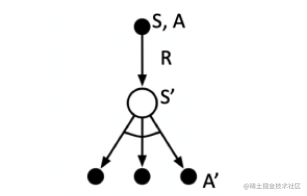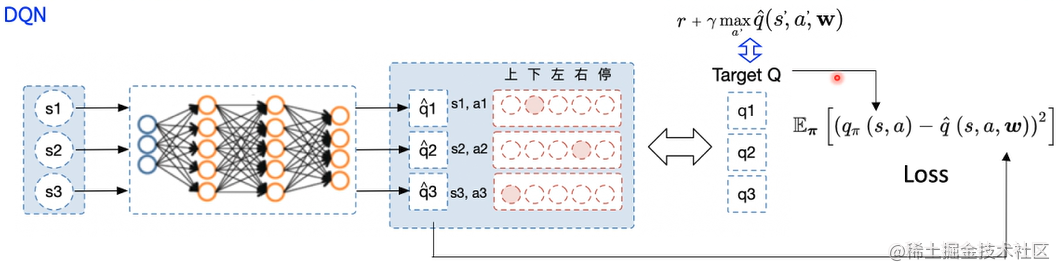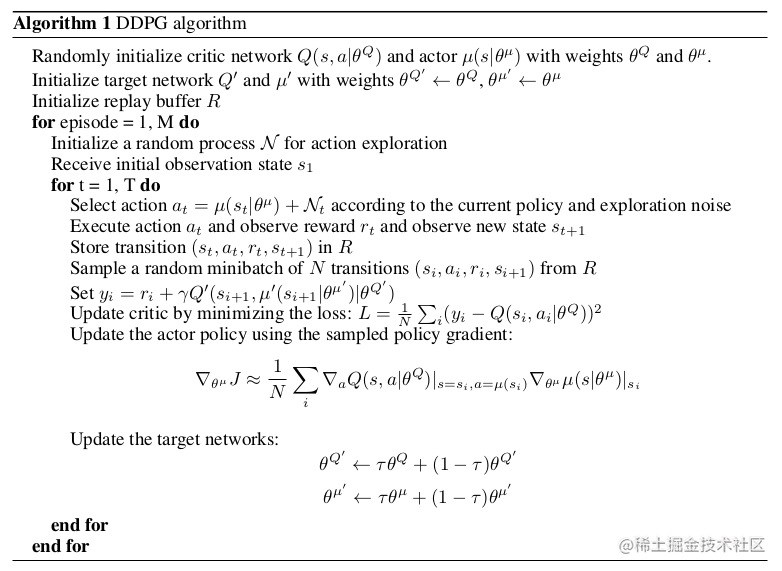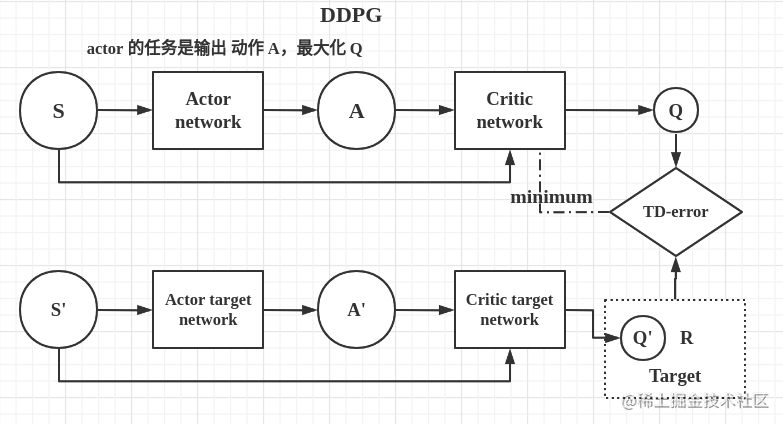一、确定性策略

$a_t = \mu(s|\theta^\mu)$

二、DDPG

1、从 Q-Learning 到 DQN

$A' = max_{a'} Q(S',a')$2、从DQN 到DDPG

$max_aQ(s,a) \approx Q(s,\mu(s|\theta))$

DDPG既然来自DQN，那当然也有经验回放与双网络，所以在 Critic 部分增加一个目标网络去计算目标 Q值，但是还需要选取动作用来估计目标Q值，由于我们有自己的Actor策略网络，用类似的做法，再增加一个Actor目标网络，所以DDPG算法一共包含了四个网络，分别是：Actor当前网络，Actor目标网络，Critic当前网络，Critic目标网络，2个Actor网络的结构相同，2个Critic网络的结构相同，这四个网络的功能如下：

• Actor当前网络：负责策略网络参数 $\theta$ 的迭代更新，负责根据当前状态 S 选择当前动作 A，用于和环境交互生成 S'和 R。
• Actor目标网络：负责根据经验回放池中采样的下一状态 S' 选择最优下一动作 A'，网络参数 $\theta$ 定期从 $\theta$ 复制。
• Critic当前网络：负责价值网络参数ww的迭代更新，负责计算负责计算当前Q值 $Q(S,A,w)$ 。目标Q 值 $y_i = R + \gamma Q'(S',A',w')$
• Critic目标网络：负责计算目标Q值中的 $Q'(S',A',w')$ 部分，网络参数 $w'$ 定期从 $w$ 复制。

$w' \leftarrow \tau w + (1-\tau)w' \\ \theta \leftarrow \tau \theta + (1-\tau)\theta'$

$A = \pi_\theta(s) + OU_{noise}$

$J(w) = \frac{1}{m}\sum_{i=1}^m(y_i - Q(S_i, A_i, w))^2$

$J(\theta) = -\frac{1}{m}\sum_{i=1}^mQ(s_i, a_i, w)$三、代码实现

1、搭建网络

Actor 网络

def get_actor(input_state_shape):
input_layer = tl.layers.Input(input_state_shape)
layer = tl.layers.Dense(n_units=64, act=tf.nn.relu)(input_layer)
layer = tl.layers.Dense(n_units=64, act=tf.nn.relu)(layer)
layer = tl.layers.Dense(n_units=action_dim, act=tf.nn.tanh)(layer)
layer = tl.layers.Lambda(lambda x: action_range * x)(layer)
return tl.models.Model(inputs=input_layer, outputs=layer)

Actor 网络输入状态 ，输出动作，注意的是，连续环境的动作一般都有一个范围，这个范围在环境中已经定以好，使用 action_bound = env.action_space.high 即可获取。

Critic 网络

def get_critic(input_state_shape, input_action_shape):
state_input = tl.layers.Input(input_state_shape)
action_input = tl.layers.Input(input_action_shape)
layer = tl.layers.Concat(1)([state_input, action_input])
layer = tl.layers.Dense(n_units=64, act=tf.nn.relu)(layer)
layer = tl.layers.Dense(n_units=64, act=tf.nn.relu)(layer)
layer = tl.layers.Dense(n_units=1, name='C_out')(layer)
return tl.models.Model(inputs=[state_input, action_input], outputs=layer)

2、主流程

for episode in range(TRAIN_EPISODES):
state = env.reset()
for step in range(MAX_STEPS):
if RENDER: env.render()
action = agent.get_action(state)
state_, reward, done, info = env.step(action)
agent.store_transition(state, action, reward, state_)

if agent.pointer > MEMORY_CAPACITY:
agent.learn()

state = state_
if done: break

def get_action(self, s, greedy=False):
a = self.actor(np.array([s], dtype=np.float32))
if greedy:
return a
return np.clip(
np.random.normal(a, self.var), -self.action_range, self.action_range)

get_action() 函数用以选取一个动作，然后与环境交互。为了更好的探索环境，我们在训练过程中为动作加入噪声，原始的DDPG作者推荐加入与时间相关的OU噪声，但是更近的结果表明高斯噪声表现地更好。由于后者更为简单，因此其更为常用。

3、网络更新

Critic 更新actions_ = self.actor_target(states_)
q_ = self.critic_target([states_, actions_])
target = rewards + GAMMA * q_
q_pred = self.critic([states, actions])
td_error = tf.losses.mean_squared_error(target, q_pred)

Actor 更新

actions = self.actor(states)
q = self.critic([states, actions])
actor_loss = -tf.reduce_mean(q)  # maximize the q

DDPG采用梯度上升法，Actor作用就是输出一个动作，这个动作输入Critic网络能得到最大的 Q 值。由于和梯度下降方向相反，所以需要在 loss 函数前面加上负号。

四、总结

DDPG通过借鉴AC的架构，在DQN算法的基础上引入了Actor网络，解决了连续控制问题，可以看做是DQN在连续问题上的改进算法。